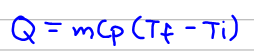# Problem: A 27.7-g sample of the radiator coolant ethylene glycol releases 688 J of heat. What was the initial temperature of the sample if the final temperature is 32.5°C (c of ethylene glycol = 2.42 J/g•K)?

###### FREE Expert Solution

Since we are given heat, with specific heat capacity and mass, we can use the formula below to solve for the initial temperature (Ti):Where:
Q = heat
m = mass
Cp = specific heat
Tf= Final temperature
Ti = Initial temperature

95% (15 ratings)###### Problem Details

A 27.7-g sample of the radiator coolant ethylene glycol releases 688 J of heat. What was the initial temperature of the sample if the final temperature is 32.5°C (c of ethylene glycol = 2.42 J/g•K)?